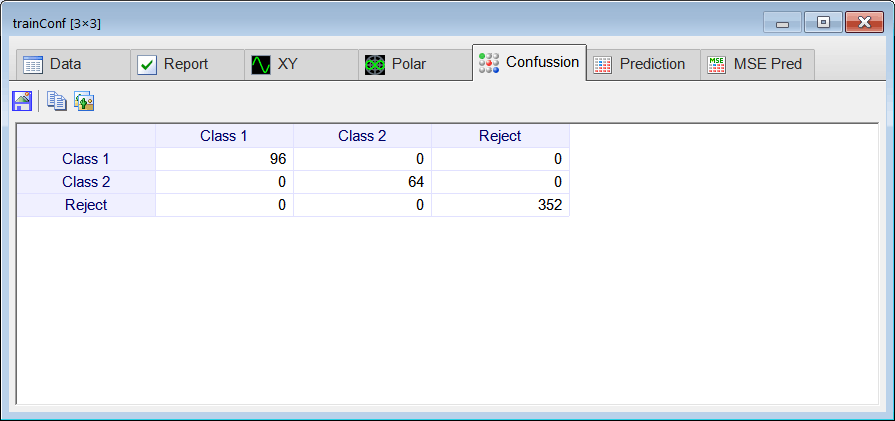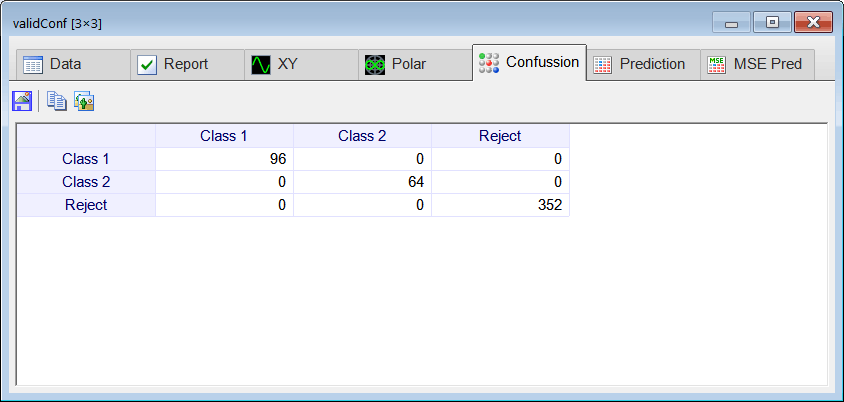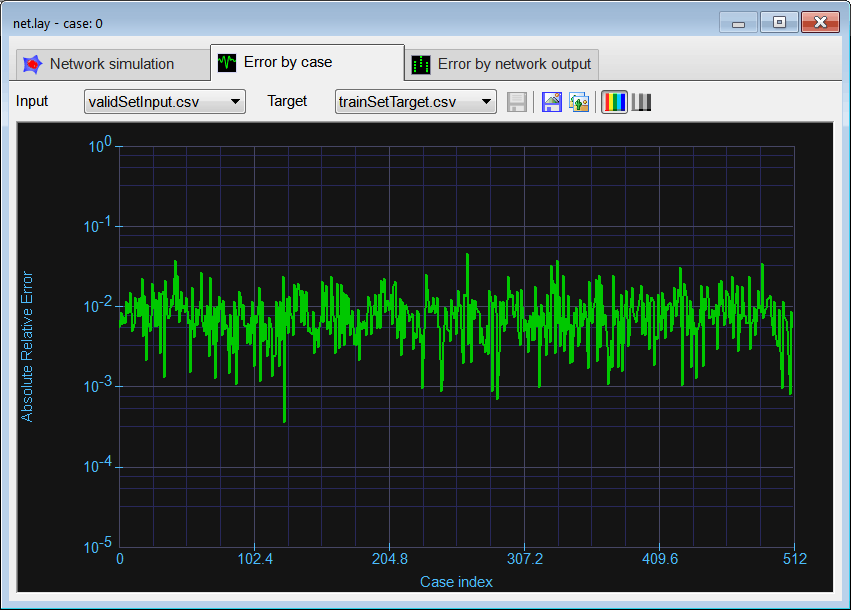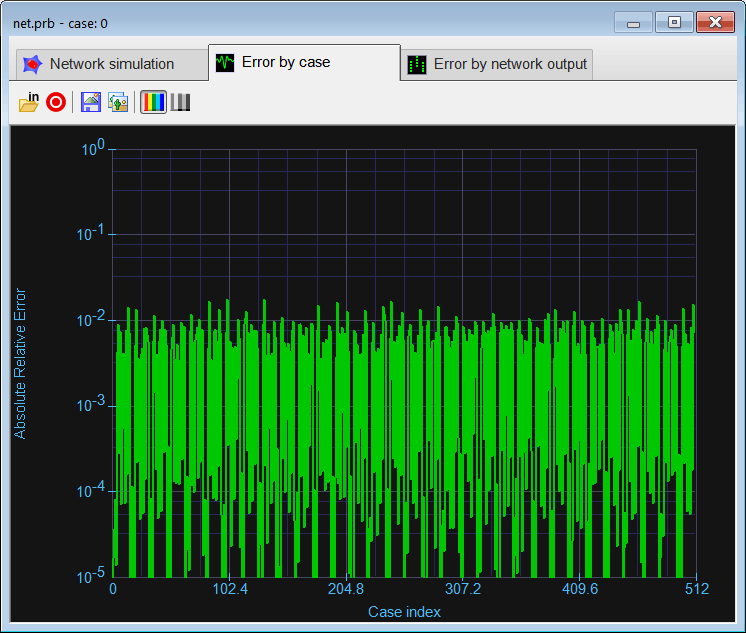# Number Classification using Probabilistic Neural Networks

 Tip One of the main differences between Multi layer ANNs and PNNs is that, you will need the training set input and training set target for training as well as for RUNNING the network.

 Problem 1 Repeat the problem in: Neural Lab > Classification > Numeric Classification using probabilistic neural networks (PNN). Create a New Project called NumProb, you may select the Probabilistic Network option. As the training set and validation set were already created, just copy the files: trainSetInput.csv, trainSetTarget.csv, validSetInput.csv and validSetTarget.csv to a new folder for this problem.

 NumProb\CheckTraining.lab //_______________________ Load the training set Matrix trainSetInput; trainSetInput.Load(); Matrix trainSetTarget; trainSetTarget.Load(); //_______________________ Load the PNN ProbNet net; net.Load(); //_______________________ Run Matrix output = net.Run(trainSetInput, trainSetTarget, trainSetInput); //_______________________ Compute the confusion matrix Matrix trainConf = ConfusionMatrix(output, trainSetTarget, 0.5); trainConf.Save(); int numErrors = toint(trainConf.GetSum()) - toint(trainConf.GetDiagonalSum());NumProb\Validation.lab //_______________________ Load the validation set Matrix validSetInput; validSetInput.Load(); Matrix trainSetTarget; trainSetTarget.Load(); //_______________________ Load the training set Matrix trainSetInput; trainSetInput.Load(); //_______________________ Load the ANN ProbNet net; net.Load(); //_______________________ Run Matrix output = net.Run(trainSetInput, trainSetTarget, validSetInput); //_______________________ Compute the confusion matrix Matrix validConf = ConfusionMatrix(output, trainSetTarget, 0.5); validConf.Save(); int numErrors = toint(validConf.GetSum()) - toint(validConf.GetDiagonalSum());Problem 2 Compare the validation performance of the PNN with the validation performance of the ANN previously trained. Observe that in a PNN, you cannot adjust the number of hidden neurons (as you can in an ANN) to improve the mse. (a) Plot the mse for case for the validation set for the ANN in the problem Neural Lab > Classification > Numeric Classification .